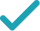Type a math problemSolve for xSteps Using the Quadratic Formula
Steps for Completing the Square
All equations of the form can be solved using the quadratic formula: . The quadratic formula gives two solutions, one when is addition and one when it is subtraction.
Subtract from both sides of the equation.
Subtracting from itself leaves .
This equation is in standard form: . Substitute for , for , and for in the quadratic formula, .
Square .
Multiply times .
The opposite of is .
Now solve the equation when is plus. Add to .
Now solve the equation when is minus. Subtract from .
The equation is now solved.
Solve for ySteps for Solving Linear Equation
Subtract from both sides.
The equation is in standard form.
Divide both sides by .
Dividing by undoes the multiplication by .
Solve for x (complex solution)Graph
x^{2}-5x+3y=20
All equations of the form ax^{2}+bx+c=0 can be solved using the quadratic formula: \frac{-b±\sqrt{b^{2}-4ac}}{2a}. The quadratic formula gives two solutions, one when ± is addition and one when it is subtraction.
x^{2}-5x+3y-20=20-20
Subtract 20 from both sides of the equation.
x^{2}-5x+3y-20=0
Subtracting 20 from itself leaves 0.
x=\frac{-\left(-5\right)±\sqrt{\left(-5\right)^{2}-4\left(3y-20\right)}}{2}
This equation is in standard form: ax^{2}+bx+c=0. Substitute 1 for a, -5 for b, and 3y-20 for c in the quadratic formula, \frac{-b±\sqrt{b^{2}-4ac}}{2a}.
x=\frac{-\left(-5\right)±\sqrt{25-4\left(3y-20\right)}}{2}
Square -5.
x=\frac{-\left(-5\right)±\sqrt{25+80-12y}}{2}
Multiply -4 times 3y-20.
x=\frac{-\left(-5\right)±\sqrt{105-12y}}{2}
x=\frac{5±\sqrt{105-12y}}{2}
The opposite of -5 is 5.
x=\frac{\sqrt{105-12y}+5}{2}
Now solve the equation x=\frac{5±\sqrt{105-12y}}{2} when ± is plus. Add 5 to \sqrt{105-12y}.
x=\frac{-\sqrt{105-12y}+5}{2}
Now solve the equation x=\frac{5±\sqrt{105-12y}}{2} when ± is minus. Subtract \sqrt{105-12y} from 5.
x=\frac{\sqrt{105-12y}+5}{2} x=\frac{-\sqrt{105-12y}+5}{2}
The equation is now solved.
x^{2}-5x+3y=20
Quadratic equations such as this one can be solved by completing the square. In order to complete the square, the equation must first be in the form x^{2}+bx=c.
x^{2}-5x+3y-3y=20-3y
Subtract 3y from both sides of the equation.
x^{2}-5x=20-3y
Subtracting 3y from itself leaves 0.
x^{2}-5x+\left(-\frac{5}{2}\right)^{2}=20-3y+\left(-\frac{5}{2}\right)^{2}
Divide -5, the coefficient of the x term, by 2 to get -\frac{5}{2}=-2.5. Then add the square of -\frac{5}{2}=-2.5 to both sides of the equation. This step makes the left hand side of the equation a perfect square.
x^{2}-5x+\frac{25}{4}=20-3y+\frac{25}{4}
Square -\frac{5}{2}=-2.5 by squaring both the numerator and the denominator of the fraction.
x^{2}-5x+\frac{25}{4}=\frac{105}{4}-3y
\left(x-\frac{5}{2}\right)^{2}=\frac{105}{4}-3y
Factor x^{2}-5x+\frac{25}{4}. In general, when x^{2}+bx+c is a perfect square, it can always be factored as \left(x+\frac{b}{2}\right)^{2}.
\sqrt{\left(x-\frac{5}{2}\right)^{2}}=\sqrt{\frac{105}{4}-3y}
Take the square root of both sides of the equation.
x-\frac{5}{2}=\frac{\sqrt{105-12y}}{2} x-\frac{5}{2}=-\frac{\sqrt{105-12y}}{2}
Simplify.
x=\frac{\sqrt{105-12y}+5}{2} x=\frac{-\sqrt{105-12y}+5}{2}
Add \frac{5}{2}=2.5 to both sides of the equation.
-5x+3y=20-x^{2}
Subtract x^{2} from both sides.
3y=20-x^{2}+5x